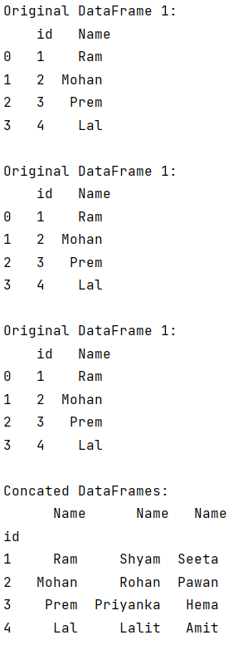# Merge a list of dataframes to create one dataframe

Given a list of pandas dataframes, we have to merge them in one dataframe.
Submitted by Pranit Sharma, on September 09, 2022

Pandas is a special tool that allows us to perform complex manipulations of data effectively and efficiently. Inside pandas, we mostly deal with a dataset in the form of DataFrame. DataFrames are 2-dimensional data structures in pandas. DataFrames consist of rows, columns, and data.

## How to merge a list of dataframes to one dataframe?

We are given a list of DataFrames, and we need to perform an operation such that all the DataFrames are merged together and result in a single DataFrame.

For this purpose, we will first create multiple DataFrames with one common column, and then we will merge them using DataFrame.merge() method.

DataFrame.merge() Syntax:

```DataFrame.merge(
right,
how='inner',
on=None,
left_on=None,
right_on=None,
left_index=False,
right_index=False,
sort=False,
suffixes=('_x', '_y'),
copy=True,
indicator=False,
validate=None
)
```

Let us understand with the help of an example,

## Python code to merge a list of dataframes to create one dataframe

```# Importing pandas package
import pandas as pd

# Creating DataFrames
df1 = pd.DataFrame({'id':[1,2,3,4],'Name':['Ram','Mohan','Prem','Lal']})
df2 = pd.DataFrame({'id':[1,2,3,4],'Name':['Shyam','Rohan','Priyanka','Lalit']})
df3 = pd.DataFrame({'id':[1,2,3,4],'Name':['Seeta','Pawan','Hema','Amit']})

# Display the DataFrame
print("Original DataFrame 1:\n",df1,"\n")
print("Original DataFrame 1:\n",df1,"\n")
print("Original DataFrame 1:\n",df1,"\n")

# encapsulating all the dfs in a list
df_list = [df1,df2,df3]

# Merging the dataframes
df = [df.set_index('id') for df in df_list]

# Display modified DataFrames
print("Concerted DataFrames:\n",pd.concat(df, axis=1))
```

Output:Reference:

What's New (MCQs)

Top Interview Coding Problems/Challenges!

Languages: » C » C++ » C++ STL » Java » Data Structure » C#.Net » Android » Kotlin » SQL
Web Technologies: » PHP » Python » JavaScript » CSS » Ajax » Node.js » Web programming/HTML
Solved programs: » C » C++ » DS » Java » C#
Aptitude que. & ans.: » C » C++ » Java » DBMS
Interview que. & ans.: » C » Embedded C » Java » SEO » HR
CS Subjects: » CS Basics » O.S. » Networks » DBMS » Embedded Systems » Cloud Computing
» Machine learning » CS Organizations » Linux » DOS
More: » Articles » Puzzles » News/Updates

© https://www.includehelp.com some rights reserved.